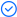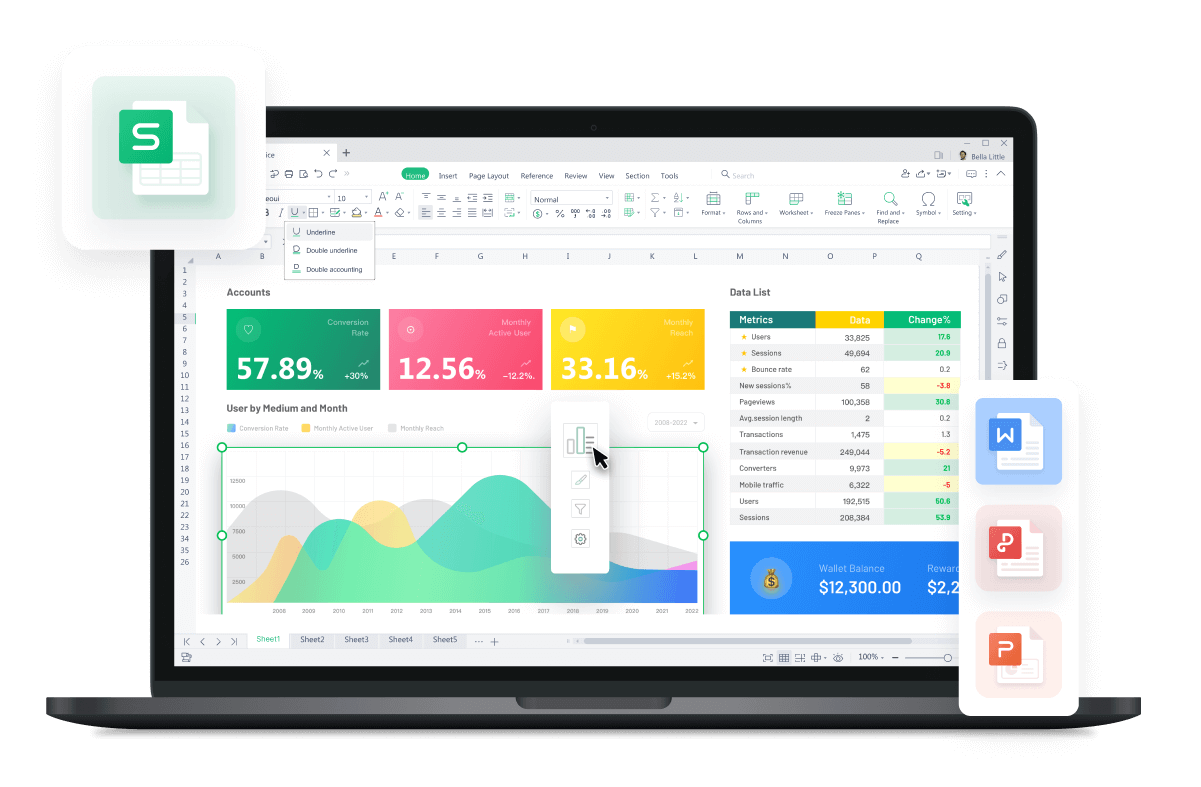WPS Office

Free All-in-One Office Suite with PDF EditorRead, edit, and convert PDFs with the powerful PDF toolkit.Microsoft-like interface, easy to use.

Windows • MacOS • Linux • iOS • Android# Text Function In Excel: How to Format Excel Codes

August 1, 2022
2.8K Views

In excel every function plays a crucial role which allows, the user, to have multiple formatting types and numbers. And we are going to help you to understand multiple ways to use format codes of text function for many purposes.

Format codes of Excel Text Function:

Our first step is to understand the purpose and the syntax of TEXT Function. And here the syntax is:

=TEXT(value, format_text)

Now, by using the TEXT function we are able to format so many values for a specific format. For where we will be needing a Text function. For instance, its example can be:

=TEXT(TODAY(),”DD/MM/YYYY”)

The output will be like this:

The date of today is in DD/MM/YYYY format, which will be 22/07/2022. Hence, using this TEXT Function we can efficiently customize our final outcome as per need or desire.

Moreover, there are many excel TEXT Functions format codes, but here you will be learning the most commonly used ones.

FORMAT CODE AND THEIR PURPOSES:

0 : it shows insignificant zeros and is a digit placeholder.

# : Another digit placeholder that works as an optional digit and is not going to display unnecessary zeros.

[ ] : it is used for creating conditional formatting.

. (period): denoted for a decimal point.

? : by leaving a space for unnecessary zeros but doesn’t let them display and is also a digit placeholder.

, : is used as a separator. As if it follows a digit placeholder. It scales the specific number by thousands.

Manage to form a custom format by combining Text and Numbers:

Let us consider having data on some vegetables and their quantity and per unit cost. Here, the total price column is the last one. And so we will be calculating the total price by using a currency symbol and text, a thousand separator, and two decimals place by using the TEXT function.

Our data set here is:

Here, the formula we will be using is mentioned below:

=Total Price &TEXT(B5*C5,\$###,###.00)

Insert this formula in cell D5.Press enter Key.Now drag it down to cell D9 and you are done having the total price.

Manage to Convert Numbers or Values to Phone numbers by using a specific format:

Now, let us consider the dataset of an individual with their cell numbers. But the numbers which are given are not properly formatted. So, we will be converting the given phone numbers into proper phone numbers by using the text function method. And we have to create phone numbers like:

555-5689

And our dataset here is given below:

And so we have to use the formula mentioned below:

=TEXT(B3,[

1. Enter the formula in cell C3.

2. Press the Enter key.

3. Drag down the format to the desired cell.

Now, you have learned various methods of using text function in excel spreadsheet.